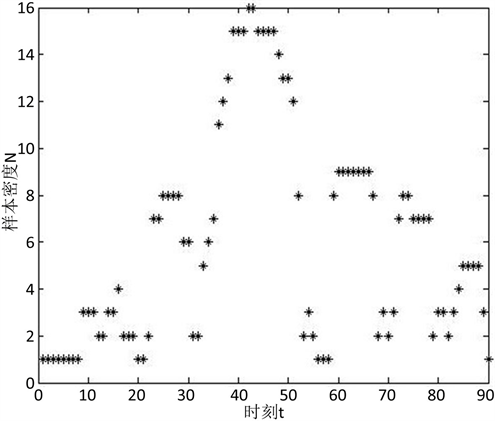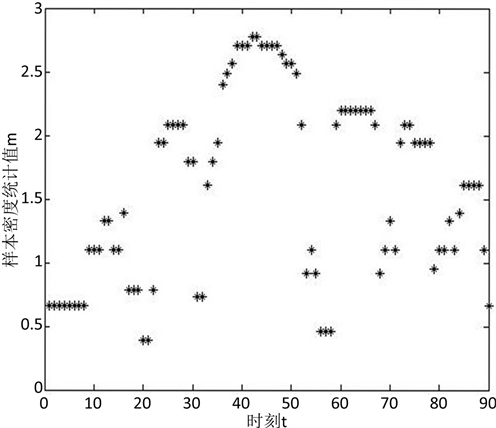# 基于DBSCAN算法的滑坡阶段划分Division of Landslide Stages Based on DBSCANAlgorithm

DOI: 10.12677/AAM.2020.92019, PDF, HTML, XML, 下载: 225  浏览: 312  科研立项经费支持

Abstract: The division of landslide stages is an important issue in the evolution of landslides. The Densi-ty-Based Spatial Clustering of Applications with Noise (DBSCAN) algorithm is used to cluster the cumulative displacement sequence data of the landslide in this paper. When the number of clus-tering clusters is greater than the number of stages, a statistic M is introduced to indicate the de-gree of the landslide changing from a stable development state to an unstable rapid deformation development state. The landslide moment corresponding to the smallest statistical value m is se-lected as the stage division point of landslide evolution and deformation. The experimental results show that it agrees with the boundary point of the actual landslide stage. It is shown that the DBSCAN algorithm can effectively divide the landslide stage accurately.

1. 引言

2. 算法介绍

2.1. DBSCAN算法的基本原理

DBSCAN算法是一种基于密度聚类的算法 ，一般假设样本的类别能通过样本的分布情况即紧密程度来确定。同属于一个类别的样本，样本之间是紧密相连的，也就是说，在某类别的任一样本局部一定有相同类别的样本存在，得到一个聚类类别，就是将密度相连的样本归为一个类别。通过将所有各组密度相连的样本划为各个不同的类别，最后就得到所有聚类的类别结果。

DBSCAN描述样本集的紧密程度是基于一组领域，参数(p, N)用来描述领域的样本分布的紧密程度。其中，p是样本的领域距离，N是样本的p领域中样本个数的阈值，(p, N)是算法初始设定的固定值，对于样本集 $D=\left\{{x}_{1},{x}_{2},\cdots ,{x}_{n}\right\}$，DBSCAN算法的具体密度描述定义如下：

1) p领域：对于 ${x}_{i}\in D$，其p领域包含样本集D与 ${x}_{j}$ 的距离不大于p的子样本集，即 $N\left({x}_{i}\right)=\left\{{x}_{j}\in D|p\left({x}_{i},{x}_{j}\right)\le p\right\}$，样本 ${x}_{i}$ 的密度记为 $N\left({x}_{i}\right)$

2) 核心对象：对于任一样本 ${x}_{i}\in D$，如果其p领域对应的密度 $N\left({x}_{i}\right)$ 至少包含N个样本，即 $N\left({x}_{i}\right)\ge N$，则 ${x}_{i}$ 是核心对象。

3) 非核心对象：对于任一样本 ${x}_{i}\in D$，如果其p领域对应的密度 $N\left({x}_{i}\right)$ 包含少于N个样本，即 $N\left({x}_{i}\right)，则 ${x}_{i}$ 是非核心对象。

4) 密度直达：如果样本 ${x}_{j}$ 在样本 ${x}_{i}$ 的p领域中，且 ${x}_{i}$ 是核心对象，则称 ${x}_{j}$${x}_{i}$ 密度直达。

5) 密度可达：对于 ${x}_{i}$${x}_{j}$，如果存在样本序列 $\left\{{x}_{{k}_{1}},{x}_{{k}_{2}},\cdots ,{x}_{{k}_{m}}\right\}$，有 ${x}_{{k}_{1}}={x}_{i}$${x}_{{k}_{m}}={x}_{j}$，且 ${x}_{{k}_{i+1}}$${x}_{{k}_{i}}$ 密度直达，则称 ${x}_{j}$${x}_{i}$ 密度可达。即，密度可达具有传递性。此时序列中的传递样本全部为核心对象。

6) 密度相连：对于 ${x}_{i}$${x}_{j}$，如果存在核心对象样本 ${x}_{k}$，使 ${x}_{i}$${x}_{j}$ 均由 ${x}_{k}$ 密度可达，则称 ${x}_{i}$${x}_{j}$ 密度相连。

2.2. DBCSCAN算法与统计量M

DBSCAN聚类算法的特点在于对聚类簇的个数未知，有可能大于我们需要划分的三个阶段，若聚类簇的个数k大于3，则需要考虑合并聚类簇，使得滑坡累积位移数据聚类为3个聚类簇。由于本文实际上为应用DBSCAN算法对滑坡的累积位移序列作阶段划分，可理解为分割问题。在累积位移序列中，聚类簇的分界点可以考虑只存在于非核心对象中，问题转化为在k-1个聚类分界点中选择出最合适的两个分界点作为滑坡的阶段划分的分割点。

$f\left({x}_{i}\right)=\left\{\begin{array}{l}{\mathrm{log}}_{2}N\left({x}_{i}\right)\text{}N\left({x}_{i}\right)\ge N\\ \underset{j=1}{\overset{2}{\sum }}\frac{N\left({x}_{i}\right)}{N\left({x}_{{i}_{j}}\right)}\text{}\text{ }N\left({x}_{i}\right) (1)

2.3. DBSCAN算法的参数确定

2.3.1. p领域的半径确定

${p}_{i}=\lambda {x}_{i}$ (2)

${\lambda }_{i}=\frac{|{x}_{i}-{x}_{i-1}|}{{x}_{i}}$ (3)

$\stackrel{¯}{\lambda }=\frac{1}{n}\underset{i=1}{\overset{n}{\sum }}{\lambda }_{i}$ (4)

2.3.2. 密度阈值N的确定

3. 滑坡实例分析Figure 1. Cumulative displacement of Xintan landslideFigure 2. Sample density of Xintan landslideFigure 3. Sample density statistics m of Xintan landslide

4. 结论

  罗文强, 李飞翱, 刘小珊, 黄丽. 多元时间序列分析的滑坡演化阶段划分[J]. 地球科学, 2016, 41(4): 711-717.  黄丽, 樊孝菊, 罗文强. 基于有序样品聚类最优二分割算法的滑坡演化阶段划分[J]. 湖北文理学院学报, 2015, 36(2): 13-16.  韩梅. 基于改进DBSCAN的复杂工业过程建模数据异常点检测研究[D]: [硕士学位论文]. 天津: 天津工业大学, 2016.  黄雯. 数据挖掘算法及其应用研究[D]: [硕士学位论文]. 南京: 南京邮电大学, 2013.  秦佳睿. DBSCAN聚类算法的改进及在数据分析系统中的应用[D]: [硕士学位论文]. 长沙: 长沙理工大学, 2017.  韩舸. 基于外因响应的分阶段滑坡位移预测模型研究[D]: [硕士学位论文]. 武汉: 中国地质大学, 2012.